Test: Fractions- 2

# Test: Fractions- 2

Test Description

## 10 Questions MCQ Test Mathematics for Class 3 | Test: Fractions- 2

Test: Fractions- 2 for Class 3 2023 is part of Mathematics for Class 3 preparation. The Test: Fractions- 2 questions and answers have been prepared according to the Class 3 exam syllabus.The Test: Fractions- 2 MCQs are made for Class 3 2023 Exam. Find important definitions, questions, notes, meanings, examples, exercises, MCQs and online tests for Test: Fractions- 2 below.
Solutions of Test: Fractions- 2 questions in English are available as part of our Mathematics for Class 3 for Class 3 & Test: Fractions- 2 solutions in Hindi for Mathematics for Class 3 course. Download more important topics, notes, lectures and mock test series for Class 3 Exam by signing up for free. Attempt Test: Fractions- 2 | 10 questions in 15 minutes | Mock test for Class 3 preparation | Free important questions MCQ to study Mathematics for Class 3 for Class 3 Exam | Download free PDF with solutions
 1 Crore+ students have signed up on EduRev. Have you?
Test: Fractions- 2 - Question 1

### How many triangles should be unshaded so that 2 / 12 of the triangles are shaded?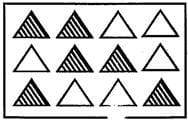Detailed Solution for Test: Fractions- 2 - Question 1

Total no. of triangles = 12
2 / 12;
So 2 triangles should be shaded so that 2 / 12 of the triangles are shaded
No. of triangles shaded = 6
No. of triangles to be unshaded = 6 - 2 = 4

Test: Fractions- 2 - Question 2

### How many more parts are to be shaded to make 1 / 2 part shaded?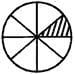Detailed Solution for Test: Fractions- 2 - Question 2

Total no. of parts = 8
No. of parts shaded = 1
No. of parts to be shaded to make 1 / 2 of the circle shaded ⇒ 1 / 2 = 4 / 8 i.e 4 parts.
No. of parts to be shaded more = 4 - 1 = 3.

Test: Fractions- 2 - Question 3

### How many squares have to be shaded to make it 1 / 8 shaded?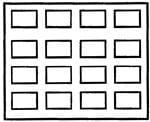Detailed Solution for Test: Fractions- 2 - Question 3

Total no. of squares = 16
No. of squares to be shaded = 2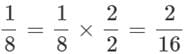Test: Fractions- 2 - Question 4

Which shows the fraction in correct order?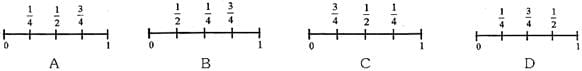Detailed Solution for Test: Fractions- 2 - Question 4

1 / 2 = 2 / 4
So, 1 / 4 < 2 / 4 < 3 / 4, hence Figure A is correct.

Test: Fractions- 2 - Question 5

The table C shows the fraction of stationery items kept in a bag. If there are 24 items in the bag. How many pencils are there?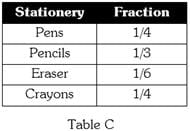Detailed Solution for Test: Fractions- 2 - Question 5

Total no. of items = 24
No. of Pencils = 1 / 3 of 24 = 1 / 3 x 24 = 8 Pencils.

Test: Fractions- 2 - Question 6

The table B shows the number of vegetable kept in a basket. What fraction of vegetables without Carrots and Onions?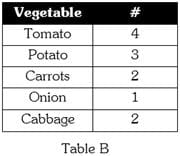Detailed Solution for Test: Fractions- 2 - Question 6

Total no. of vegetables = 12
No. of vegetables that care not carrots and onions = 12 - (4 + 3 + 2) = 3.
Fraction of veg that are not carrots onions = 3 / 12 = 14

Test: Fractions- 2 - Question 7

What fraction of the shapes are triangles?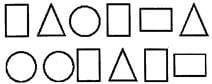Detailed Solution for Test: Fractions- 2 - Question 7

Total no. of shapes = 12
No. of triangles = 3
Fraction of triangles = 3 / 12.

Test: Fractions- 2 - Question 8

What is the missing number that completes the pattern of fraction?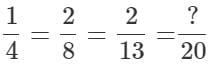Detailed Solution for Test: Fractions- 2 - Question 8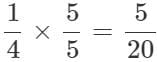Test: Fractions- 2 - Question 9

Which number makes the fraction equal?

Detailed Solution for Test: Fractions- 2 - Question 9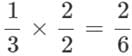So,? = 1

Test: Fractions- 2 - Question 10

Nisha got 4 pastries from a bakery. She served 2 of them to the guest's and kept the remaining in the refrigerator. What fraction of pastries are kept in the refrigerator?

Detailed Solution for Test: Fractions- 2 - Question 10

No. of pastries kept in the refrigerator = 4 - 2 = 2
Fraction of pastries kept in the refrigerator = 2 / 4 = 1 / 2.

## Mathematics for Class 3

15 videos|53 docs|23 tests
 Use Code STAYHOME200 and get INR 200 additional OFF Use Coupon Code
Information about Test: Fractions- 2 Page
In this test you can find the Exam questions for Test: Fractions- 2 solved & explained in the simplest way possible. Besides giving Questions and answers for Test: Fractions- 2, EduRev gives you an ample number of Online tests for practice

## Mathematics for Class 3

15 videos|53 docs|23 tests